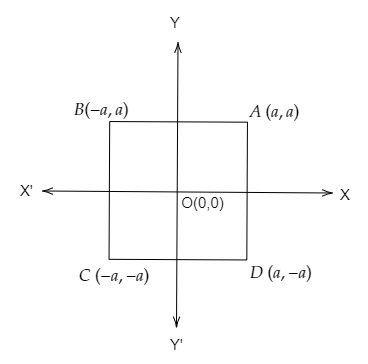# Let ABCD be a square of side 2a. Find the coordinates of the vertices of this square whenThe centre of the square is at the origin and coordinate axes are parallel to the sides AB and AD respectively.

Given:

ABCD is a square of side $2a$. The centre of the square is at the origin and coordinate axes are parallel to the sides AB and AD respectively.

To do:

We have to find the coordinates of the vertices of the square.

Solution:

The centre of the square is at origin $(0, 0)$ and co-ordinate axes are parallel to the sides AB and AD respectively.
The co-ordinates of A, B, C and D are $(a, a), (-a, a), (-a, -a)$ and $(a, -a)$ as shown in the figure given below:Therefore, the coordinates of the vertices of the square are $A\ (a,a), B\ (-a,a), C\ (-a,-a)$ and $D\ (a,-a)$.